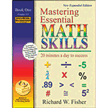#### Subtracting Mixed Numbers and Whole NumbersHow do you subtract mixed numbers from whole numbers? Uh? That’s what I thought. This is a commonly missed fraction problem. Once, you read this article your “uh” will change to “ah ha!”

I. Subtract a Whole Number from a Mixed Number

II. Subtract a Mixed Number from a Whole Number

The steps remain the same
1. Subtract the fractions
2. Subtract the whole numbers

I. Subtract a Whole Number from a Mixed Number

Example: 16 3/8 - 4 = ?

1. Subtract fractions: Well there’s only one fraction, and it happens to be the first number or on top (if you wrote the problem vertically). So, it’s the same as saying 3/8 – 0 = ? Any number minus zero equals itself. Therefore, 3/8 - 0 = 3/8

2. Subtract the whole numbers: 16 - 4 = 12

In summary: 16 3/8 - 4 = 12 3/8

Simple enough? Let us continue.

Now, the next type of problem is frequently missed. So pay close attention!

II. Subtract a Mixed Number from a Whole Number

Example: 15 - 6 5/8 = ?

1. Subtract fractions: There’s only one fraction, BUT the fraction is mentioned second or on the bottom (if you wrote the problem vertically).
So, it’s the same as saying 0 - 5/8 = ? (Houston, we have a problem! ) It’s impossible to take anything away from zero.

So, what do you do when something catches you off guard? You, regroup. Perhaps you change clothes or change your hair. You may look a little differently, but you are still the same person.

Likewise, the number, 15, must regroup to handle this problem. It will change its appearance by decreasing by one. Then, take that one and change it into a fraction by using the existing fraction’s denominator. Stop. Think; and, ask yourself how you represent one as a fraction using the denominator, 8.
Yes! 8 / 8 . Thus, the number, 15, will become a mixed number. Remember, it’s the same number with a different look. The new look is as follows: 14 8/8. (14 plus 1 equals 15) Let’s restate the problem below.

14 8/8 - 6 5/8 = ?

Now, you can handle this problem with ease.

Subtract the fractions: 8/8 - 5/8 = 3/8

2. Subtract the whole numbers: 14 – 6 = 8

Therefore, 14 8/8 - 6 5/8 = 8 3/8.

Note: The principle you learned here applies to life. If something catches you off guard, step back, regroup, and handle your business. You can do it!

So, practice! You may purchase a book with short concise practice lessons below from
Christianbook.com HomeMastering Essential Math Skills, Revised Edition: Book One Produce motivated and confident math students with this innovative, easy-to-use system of teaching basic math! Each daily lesson contains five key components: two speed drills, review exercises, Helpful Hints, new material and a daily word problem. Lessons are not rigid however; teachers have the flexibility to modify lesson plans while structure and a basic framework are still provided. Eight chapters cover whole numbers, fractions, decimals, percentages, integers, geometry, charts, graphs and problem solving. Help your students really master the essential math skills they'll need throughout their lives with enthusiasm and consistency. Answer key and final review included. 142 pages, softcover. For Grades 4-5. Book 1.

Subtracting Mixed Numbers
Subtracting Like and Unlike Denominators
GCF and LCM by Prime FactorizationRelated Articles
Editor's Picks Articles
Top Ten Articles
Previous Features
Site Map